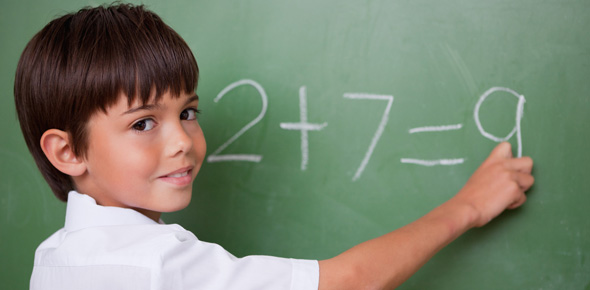# Eii Studyhelper 06

17 Questions | Total Attempts: 52SettingsThis is intended for DLSZ students. The questions are about odd and even numbers, counting money, reading and writing roman numerals, addition, properties of addition and addition of whole numbers.

Related Topics
• 1.
There are 6 boxes.  Each box has 50 toys.  How many toys are in 6 boxes?
• A.

250

• B.

300

• C.

350

• 2.
In writiing Roman numerals, which symbol can be repeated up to 3 times in succession?
• A.

D and L

• B.

I and X

• C.

V and L

• 3.
Which of the following numbers (51, 90, 55, 38) are even?
• A.

90 and 38

• B.

51 and 95

• C.

51 and 90

• 4.
Which are parts of an addition sentence?
• A.

• B.

• C.

• 5.
Which property of addition states that the order of the addends does not affect the sum?
• A.

Commutative Property

• B.

Associative Property

• C.

Identity Property

• 6.
What is the sum of 36 and and 52?
• A.

88

• B.

586

• C.

412

• 7.
Arielle's alarm clock ring every 3 hours.  It rang last at 3 o'clock in the morning.  WIll it ring at 12 o'clock?
• A.

Yes

• B.

No

• 8.
What are the two odd numbers before 867?
• A.

863 and 865

• B.

863 and 866

• C.

865 and 866

• 9.
How much is 2 - 10 peso and 3 - 5 peso?
• A.

35 pesos

• B.

40 pesos

• C.

45 pesos

• 10.
How much is 1 - 1,000 peso, 2 - 100, 2 - 50 peso?
• A.

1,200

• B.

1,300

• C.

1,400

• 11.
Which Roman numeral is written correctly?
• A.

ID

• B.

CD

• C.

VC

• 12.
What is the sum of 34 and 364?
• A.

398

• B.

604

• C.

380

• 13.
How many even numbers are there in the series "23, 42, 27, 44, 68, 33, 56?
• A.

4

• B.

5

• C.

6

• 14.
What is CMIX in Hindu-Arabic numeral?
• A.

1,009

• B.

909

• C.

999

• 15.
Mark bought a half gallon of oil and a can of cheese balls for 498 pesos and 89 pesos.  Which addition sentence fits this story?
• A.

498 + 89 = 587

• B.

498 + 89 = 1,478

• C.

498 + 89 = 409

• 16.
Check all odd numbers between 1,010 and 1,020.
• A.

1,011

• B.

1,013

• C.

1,015

• D.

1,017

• E.

1,019

• F.

1,014

• G.

1,018

• H.

1,016

• 17.
What are the missing Roman numerals in the series "XL, ___, LX, LXX, LXXX, ___
• A.

XXL and X

• B.

XXL and C

• C.

L and XC